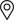Find Locally

## Convert mAh to Watt Hours

Insert milliamp hour (mAh) and voltage (V) and click on Calculate to obtain Watt hours (Wh).
mAh
V
Wh
Formula is (mAh)*(V)/1000 = (Wh). For example, if you have a 300mAh battery rated at 5V, the power is 300mAh * 5V / 1000 = 1.5Wh.

## Convert Watt Hours to mAh

Insert Watts-hour (Wh) and voltage (V) and click on Calculate to obtain milliamp-hours (mAh).
Wh
V
mAh
Formula is (Wh)*1000/(V) =(mAh). For example, if you have a 1.5Wh battery rated at 5V, the power is 1.5Wh * 1000 / 5V = 300mAh.